# 一建-第三章-建设项目总投资

80
|
20

## 相关模板推荐

•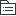## 建设项目总投资 —— 作品大纲

•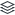生产性建设项目总投资
• 建设投资
• 设备及工器具购置费
•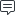积极费用
• 国产标准设备原价
• 出厂价
• 国产非标准设备原价
• 子主题
• 进口设备抵岸价
•抵达买方港口或车站且交完税
• 内陆交货
• 在卖方地完成交货
• 目的地交货
• 在买方地完成交货，费用和风险以目的地约定交货点为界线
• 装运港交货
• 买方责任：租船订舱交运费，船期船名告卖方，办理保险及交付保险费，目的港进口收货手续，承担装船后一切费用和风险，接受卖方有关装运单据，按合同支付货款
• 卖方责任：出口手续，货物装货前一切费用和风险，出口国政府或有关方面签发的证件，负责提供有关装运单据
•交货方式
• 进口设备抵岸价的构成
• 货价
• 离岸价*汇率
• 国外运费
• 离岸价*运费率 或 运量*单位运价
• 国外保险费
• 银行服务费
• 离岸价*汇率*银行服务费率
• 银行
• 外贸手续费
• （离岸价+国外运费+国外运输保险费）*手续费率*汇率
• 进口关税
• 到岸价*汇率*进口税率
•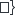海关收取
• 消费税
• 增值税
• （到岸价*汇率+进口关税+消费税）*增值税率
•海关代收
• 设备运杂费
• 设备原价（进口设备抵岸价）*设备运杂费率
• 工器具及生产家具购置费
•生产家具为施工用
• 设备购置费*定额费率
• 建筑安装工程费
• 按要素分
• 人工费
• 奖金
• 加班加点工资
• 津贴、补贴
• 特殊情况下支付的工资
• 计时工资或计件工资
•奖加贴殊计
• 材料费
• 材料原价
• 采购及保管费
• 运输损耗费
• 运杂费
•原购耗运
• 材料单价=[（材料原价+运杂费）*（1+材料损耗率）]*(1+采购保管费率）
• 工程设备单价=（设备原价+运杂费）*（1+采购保管费率）
• 施工机具使用费
• 施工机械使用费
• 折旧费
• 大修理费
• 经常修理费
• 安拆费及场外运费
• 燃料动力费
• 人工费
• 税费
•折大常安燃入税
• 机械台班单价=折+大+常+安+燃+入+税
• 仪器仪表使用费
• 企业管理费
• 管理人员工资
• 办公费
• 差旅交通费
• 固定资产使用费
• 工具用具使用费
• 劳动保险和职工福利费
• 劳动保护费
• 检验试验费
• 工会经费
• 职工教育经费
• 财产保险费
• 财务费
• 税金
• 城市维护建设税
• 教育费附加
• 地方教育附加
• 其他
•管办差固工，保护试工，教财务税
• 利润
• 规费
• 五险一金
• 养老保险
• 医疗保险
• 失业保险
•单位+个人缴纳
• 生育保险
• 工伤保险
•单位缴纳
• 住房公积金
• 工程排污费
• 按规定标准缴纳，按实计取列入
• 增值税
• 应纳税额=当期销项税额-当期进项税额=销项额*税率-进项税额*扣除率
• 一般计税方法
• 税前造价*税率
•人材机管利规且不包含增值税可抵扣进项税额的价格
• 简易计税方法
• 税前造价*3%
•人材机管利规均包含增值税进项税额的含税价
• 按造价分
• 分部分项工程
• 措施项目费
• 大型机械进出场及安拆费
• 脚手架工程费
• 特殊地区施工增加费
• 工程定位复测费
• 冬雨季施工增加费
• 夜间施工增加费
• 二次搬运费
• 已完工程及设备保护费
• 安全文明施工费
•不可计量
•大脚特工，冬夜二已安
• 其他项目费
• 暂列金额
•施工中，工程变更合同调整索赔现场签证确认工程价跨调整
• 计日工
• 总承包服务费
• 规费
• 五险一金
• 工程排污费
• 增值税
•工程费用
• 工程建设其他费用（建设单位）
• 土地使用费和其他补偿费
• 农用土地征用费
• 国有土地使用费
• 子主题
• 预备资金
• 基本预备费
• =(工程费用+工程建设其他费）*基本预备费率
• 价差预备费
• 资金措施费
• 当年本金算一般，往年本利权计算
• 铺底流动资金
• 流动资金金额=各种费用基数（年营业收入年经营成本或年产量）*相应的流动资金所占比例（或占营运资金的数额）
• 流动资金=流动资产-流动负债
• 流动资产=应收账款+预付账款+存货+库存现金
• 子主题
• 流动负债=应付账款+预收账款
•非生产性建设项目总投资
• 建设投资
•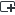浮动主题